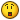## Recommended Posts

how can i count inside the loop below in hex so that the test.txt file that is written contains

mappath00=computer\share

mappath01=computer\share

mappath02=computer\share

mappath03=computer\share

mappath04=computer\share

mappath05=computer\share

mappath06=computer\share

mappath07=computer\share

mappath08=computer\share

mappath09=computer\share

mappath0a=computer\share

mappath0b=computer\share

you will need to put the attached file with the script when it runs to see what I mean!

#include<Array.au3>

#include<File.au3>

#include <String.au3>

#NoTrayIcon

filedelete ("test.txt")

If @error Then

MsgBox(4096, "", "~netfldr.tmp missing!")

Else

For \$i = 1 To \$var

\$var2=StringTrimLeft ( \$var[\$i], 2 )

FileWriteLine ( "test.txt", "mappath" & \$var & "=" & \$var2 )

MsgBox(4096, "", \$var2)

Next

EndIf

##### Share on other sites

hmmmm

so you want a hex counting function?

Edit: If so try creating a global var at the beggining that equals zero. Then create a func that converts it to decimal and adds one and then converts it back the hex. I would have made it but that would ruin the fun for you.Hint: I believe there are built in functions for converting things to hex and decimal.

Edited by fear1313

[center][/center]Working on the next big thing.Currently Playing: Halo 4, League of LegendsXBL GT: iRememberYhslaw

##### Share on other sites

hmmmm

so you want a hex counting function?

see above

i need each line to contain a two digit hex number

at the moment that script writes out this

mappath3=qserver\c\$

mappath3=Qserver\e\$

mappath3=qserver\d\$

mappath3=qserver\c\$

mappath3=Qserver\e\$

mappath3=qserver\d\$

mappath3=qserver\c\$

mappath3=Qserver\e\$

mappath3=qserver\d\$

mappath3=qserver\c\$

mappath3=Qserver\e\$

mappath3=qserver\d\$

where it should be

mappath00=qserver\c\$

mappath01=Qserver\e\$

mappath02=qserver\d\$

mappath03=qserver\c\$

mappath04=Qserver\e\$

mappath05=qserver\d\$

mappath06=qserver\c\$

mappath07=Qserver\e\$

mappath08=qserver\d\$

mappath09=qserver\c\$

mappath00=Qserver\e\$

mappath0A=qserver\d\$

(and so on (hex in caps) )

##### Share on other sites

i dont see an attached file to read from

8)whoops!

##### Share on other sites

um... i am attaching it.. but it wont do it!

so here it is, save it as ~netfldr.tmp

[\\qserver\c\$]

Name=\\qserver\c\$

User=

[\\Qserver\e\$]

Name=\\Qserver\e\$

User=

[\\qserver\d\$]

Name=\\qserver\d\$

##### Share on other sites

um... i am attaching it.. but it wont do it!

so here it is, save it as ~netfldr.tmp

[\\qserver\c\$]

Name=\\qserver\c\$

User=

[\\Qserver\e\$]

Name=\\Qserver\e\$

User=

[\\qserver\d\$]

Name=\\qserver\d\$

here is the answer for anyone else struggling!

#include<Array.au3>

#include<File.au3>

#include <String.au3>

#NoTrayIcon

filedelete ("test.txt")

If @error Then

MsgBox(4096, "", "~netfldr.tmp missing!")

Else

For \$i = 1 To \$var

\$var2=StringTrimLeft ( \$var[\$i], 2 )

\$tmp = \$i - 1

\$result = Hex(\$tmp, 2)

FileWriteLine ( "test.txt", "set mappath" & \$result & "=" & \$var2 )

; MsgBox(4096, "", \$var2)

Next

EndIf

## Create an account

Register a new account

• ### Recently Browsing   0 members

×

• Wiki

• Back

• #### Beta

• Git
• FAQ
• Our Picks
×
• Create New...